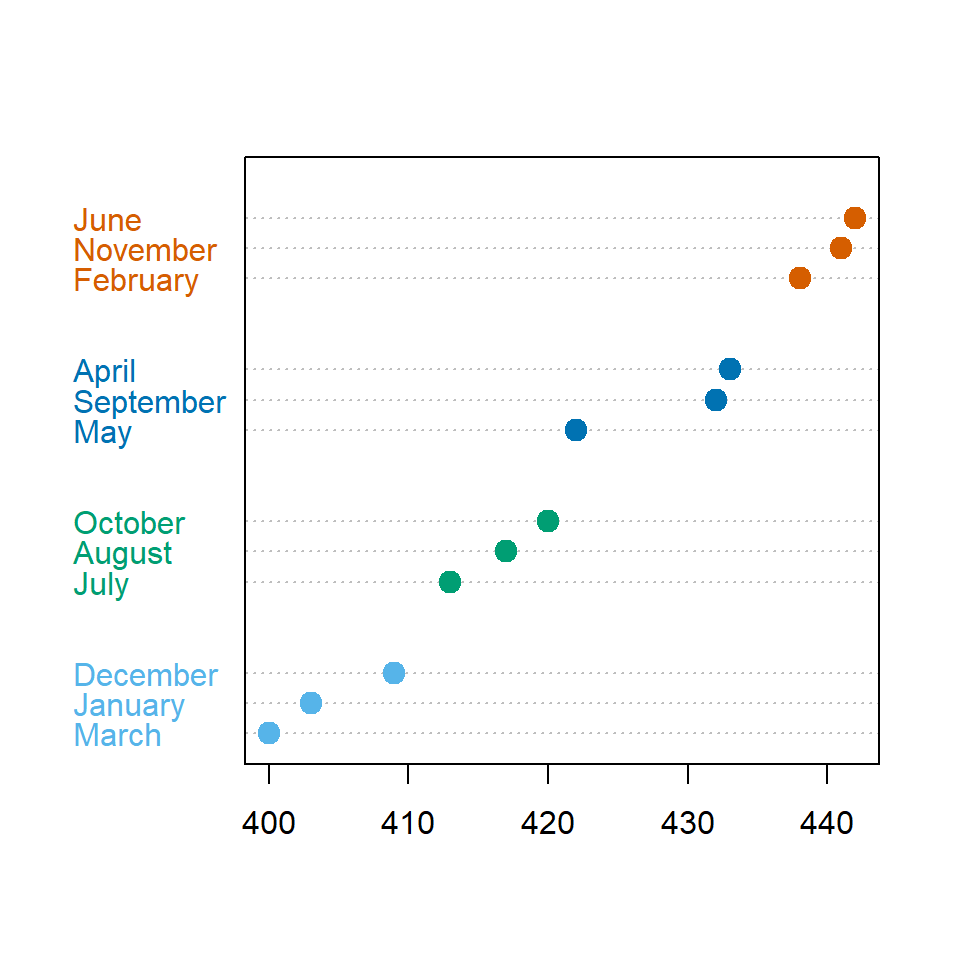# Cleveland dot plot in R

## Sample data

The following variables represent the quantity sold of a product every month of the year, the month and the quarter of the year for each month.

``````# Sample dataset
set.seed(1)
sold <- sample(400:450, 12)
month <- month.name
quarter <- c(rep(1, 3), rep(2, 3),
rep(3, 3), rep(4, 3))``````

## Basic dot plot with `dotchart` function

Basic dot chart

A dot plot can be created with the `dotchart` function from base R graphics. You can pass a vector of numeric values to the function to create the most basic chart.

``dotchart(sold)``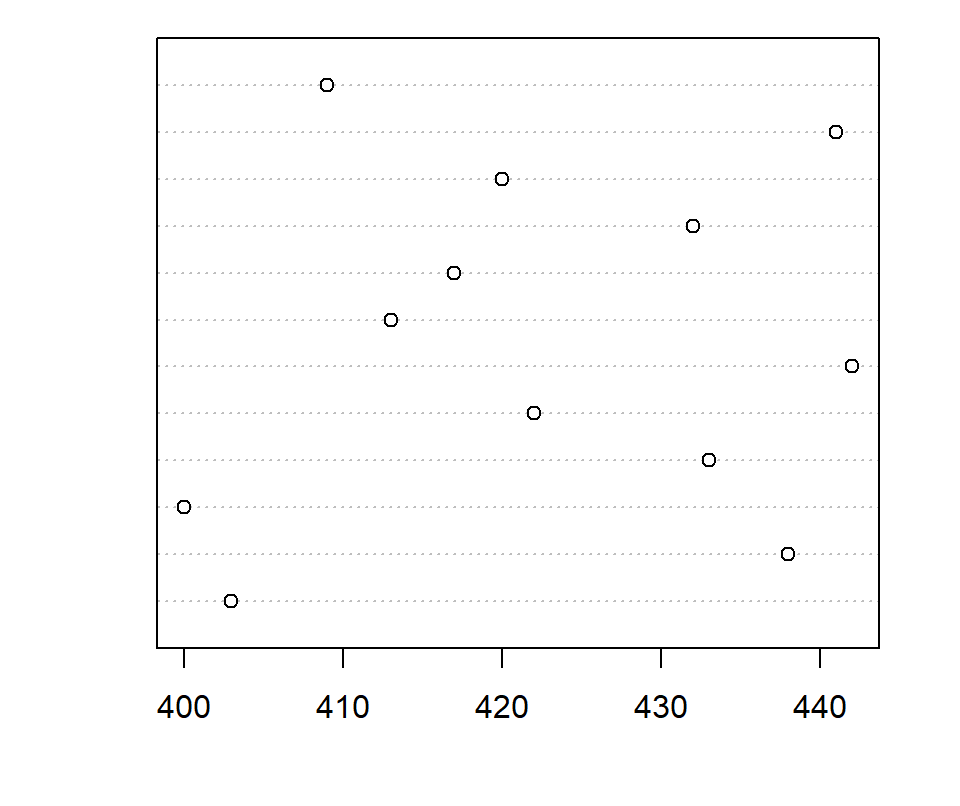Customization

There are several arguments that can be customized, such as `pch` to change the plotting character symbol, `col` to change the color of the symbols, `pt.cex` for the size of the symbols and `fram.plot`, which is a logical determining whether to draw a box around the plot or not.

``````dotchart(sold,
pch = 19,             # Symbol
col = hcl.colors(12), # Colors
pt.cex = 1.5,         # Symbol size
frame.plot = TRUE)    # Plot frame``````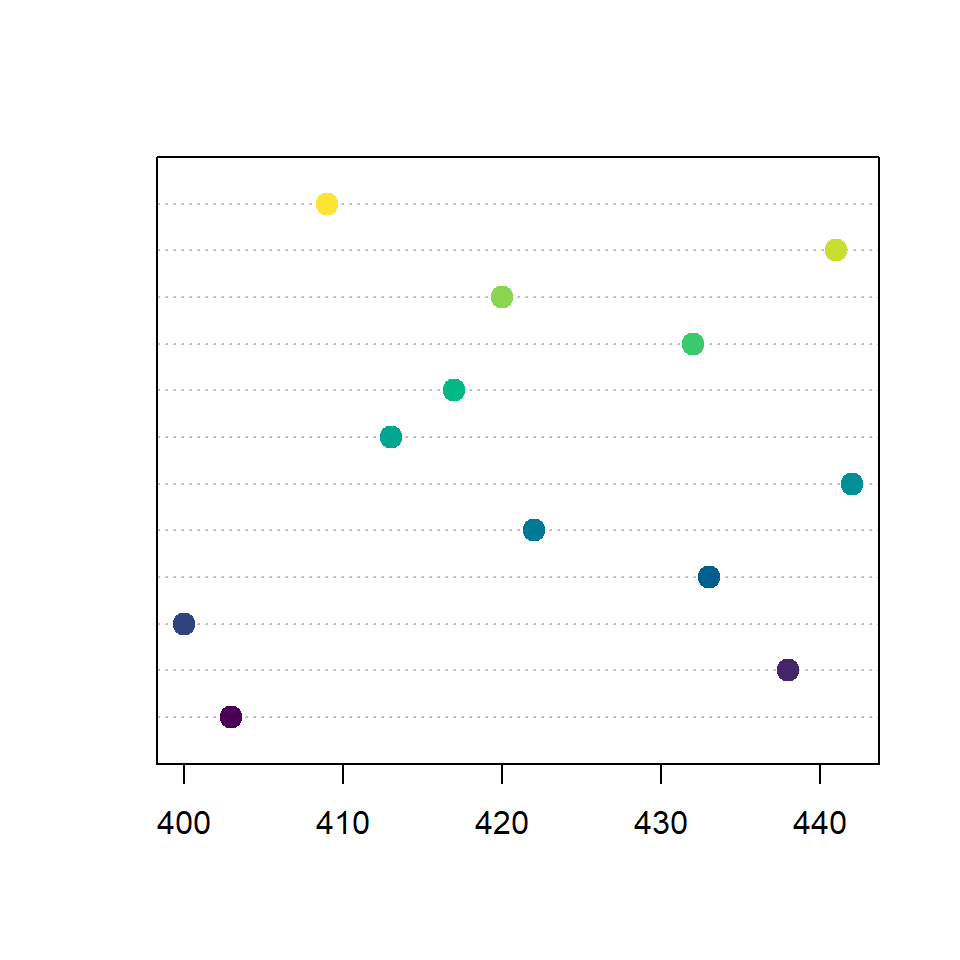Labeling the observations

You can label each point with the `labels` argument. In this example we are passing the names of the months to represent each observation.

``````dotchart(sold,
pch = 19,
col = 4,
pt.cex = 1.5,
labels = month)``````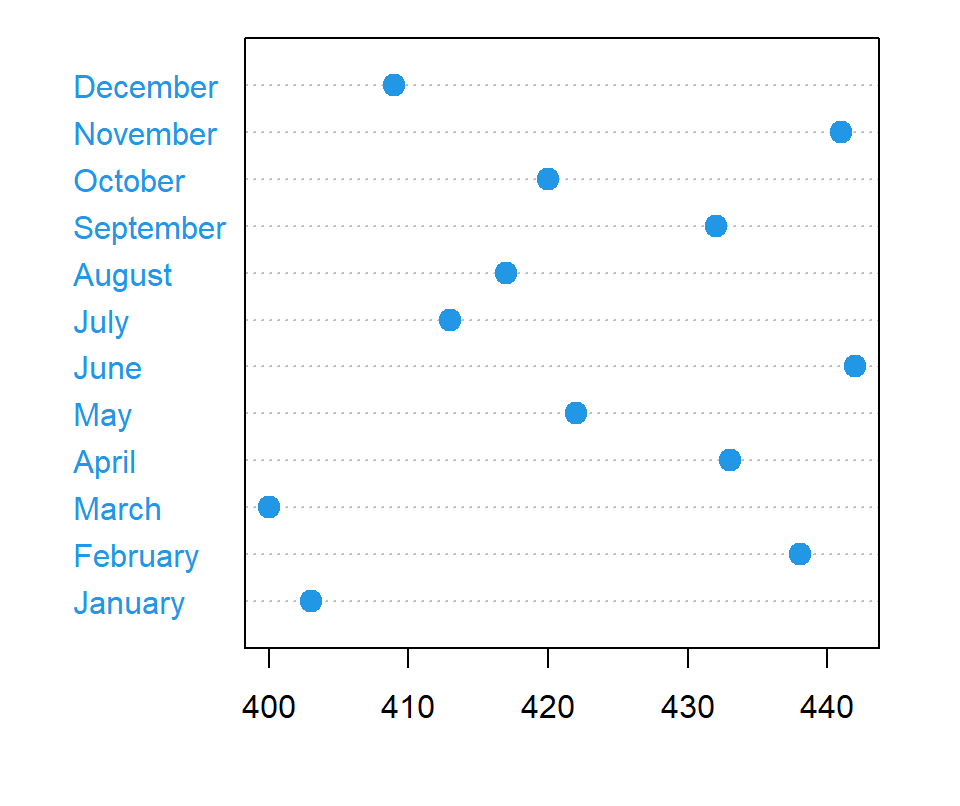## Dot chart by group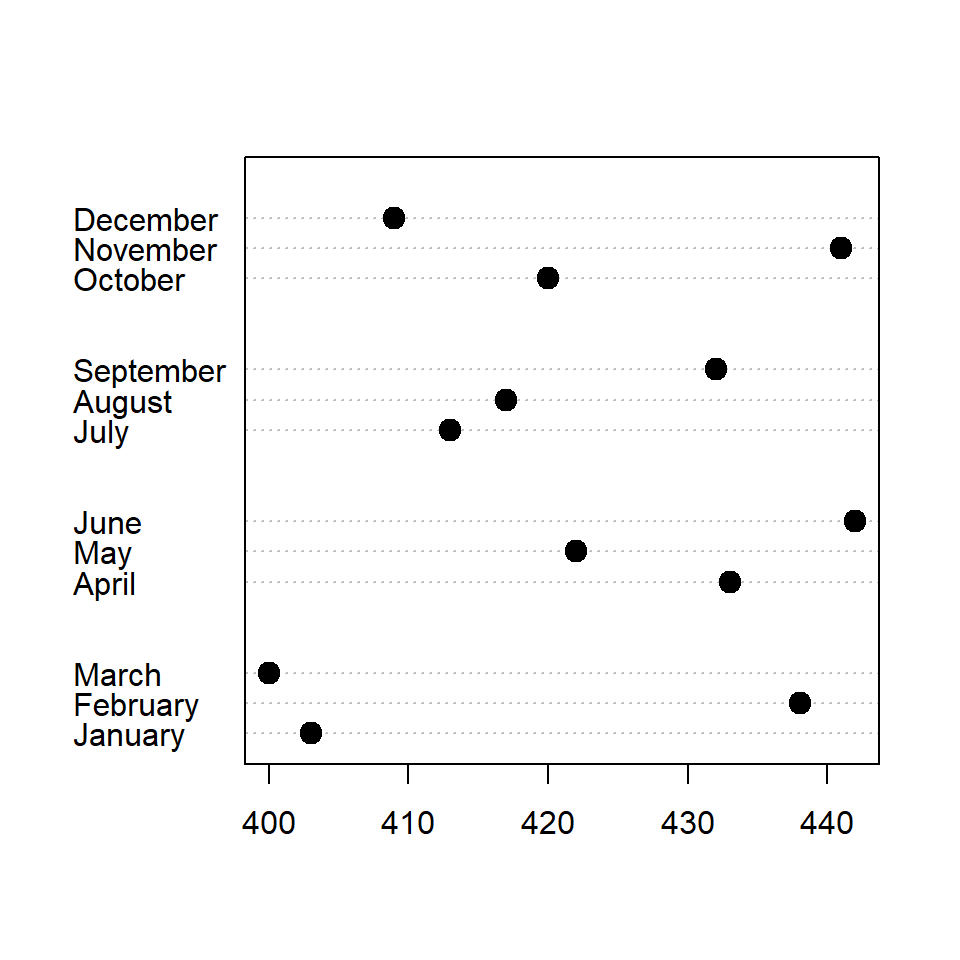Specifying groups

Given a variable representing groups it is possible to group the elements. In the following example we are passing the `quarter` variable to the `groups` argument to group the months by quarter.

``````dotchart(sold,
pch = 19, pt.cex = 1.5,
labels = month,
groups = rev(quarter))``````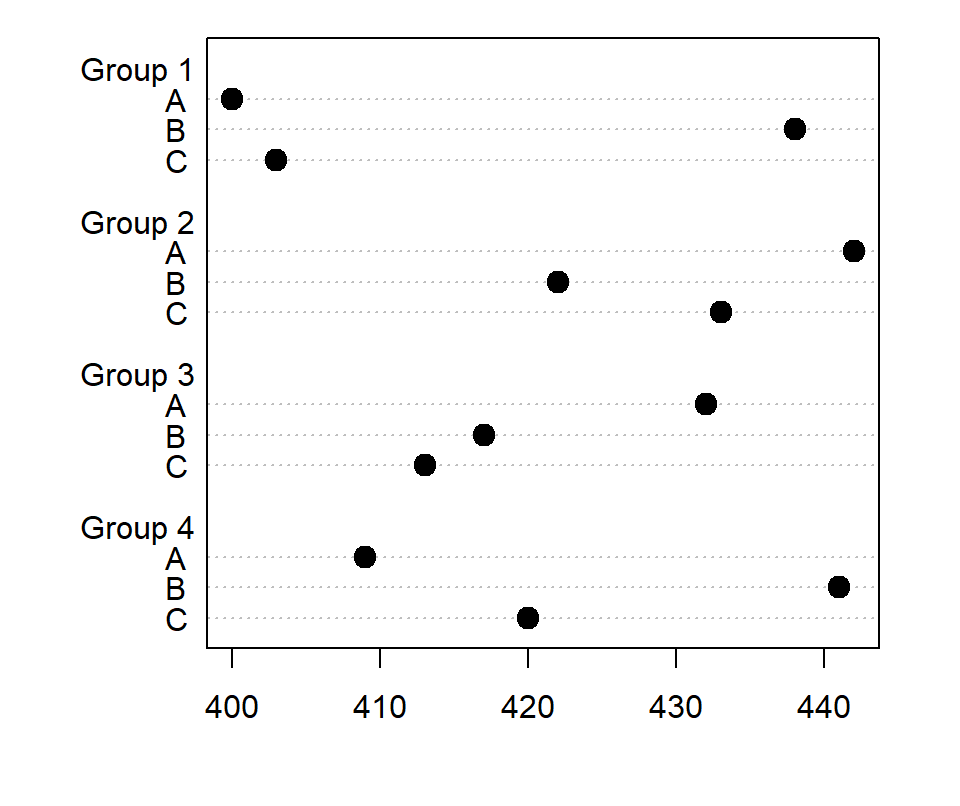Matrix as input

Note that the `dotchart` function also allows passing a matrix. In this scenario the groups will be the columns of the matrix by default and the rows the observations for each group.

``````# Matrix from the data
m <- matrix(sold, ncol = 4)
colnames(m) <- c("G1", "G2", "G3", "G4")
rownames(m) <- LETTERS[3:1]

dotchart(m, pch = 19, pt.cex = 1.5)``````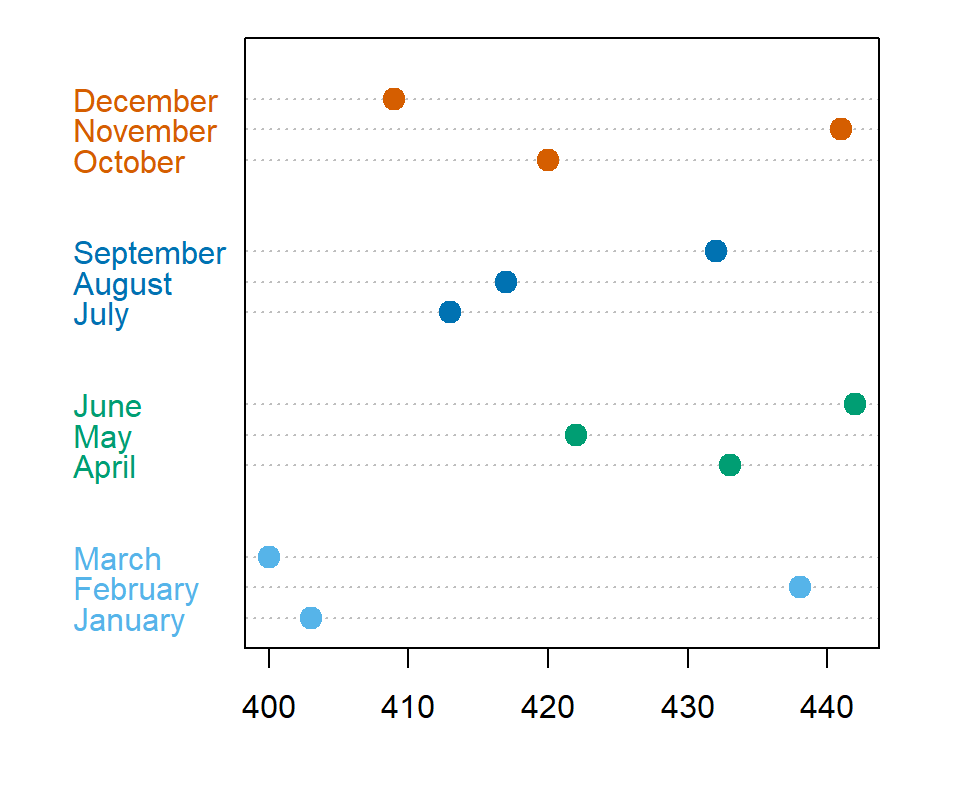Color by group

If you have passed a grouping variable to `groups` you can also customize the colors for each group, passing a vector of the same length of the input data to the `color` argument.

``````# Colors for each group
cols <- c(rep("#56B4E9", 3), rep("#009E73", 3),
rep("#0072B2", 3), rep("#D55E00", 3))

dotchart(sold,
pch = 19, pt.cex = 1.5,
labels = month,
groups = rev(quarter),
color = cols)``````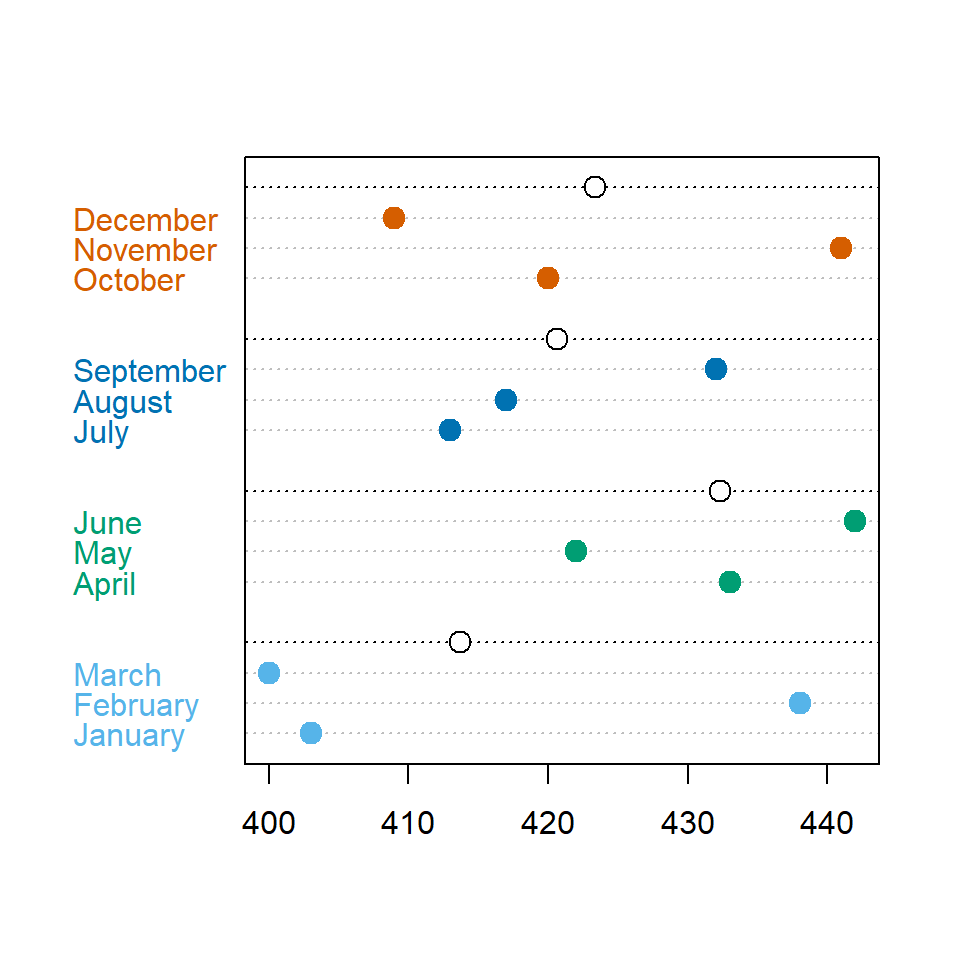Summary for each group

The `gdata` argument allows passing some measure for the groups, such as a statistical summary. In the example below we are calculating the mean of sells by quarter.

``````# Colors for each group
cols <- c(rep("red", 3), rep("blue", 3),
rep("green", 3), rep("orange", 3))

dotchart(sold,
pch = 19, pt.cex = 1.5,
labels = month,
groups = rev(quarter),
color = cols,
gdata = rev(tapply(sold, quarter, mean)))``````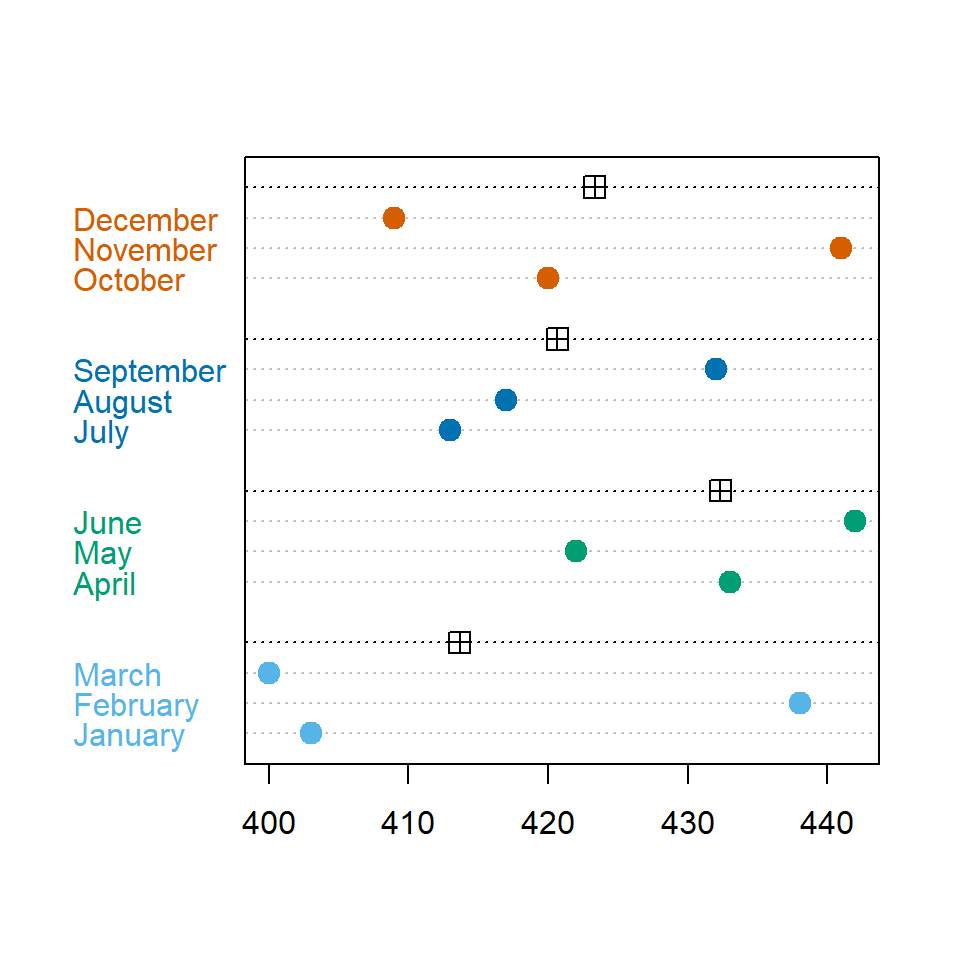The new points added with `gdata` can be customized with `gpch` and `gcolor` arguments, to modify the pch symbol and the color of the new points, respectively.

``````# Colors for each group
cols <- c(rep("#56B4E9", 3), rep("#009E73", 3),
rep("#0072B2", 3), rep("#D55E00", 3))

dotchart(sold,
pch = 19, pt.cex = 1.5,
labels = month,
groups = rev(quarter),
color = cols,
gdata = rev(tapply(sold, quarter, mean)),
gpch = 12,
gcolor = 1)``````

## Order the values

Finally, note that you can order the values and the labels based on the variable or other measure. In the following example we are ordering the months based on the amount of sales.

``````# Colors for each group
cols <- c(rep("#56B4E9", 3), rep("#009E73", 3),
rep("#0072B2", 3), rep("#D55E00", 3))

dotchart(sort(sold),
labels = month[order(sold)],
pch = 19, pt.cex = 1.5,
groups = rev(quarter),
color = cols)``````